Create a new printableGeometry
Math Worksheets

Sample - Click above to make a new math worksheet (PDF).
 Name _____________________________Date ___________________
Lines and Angles
Find the measure of the angle.

1.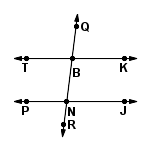TK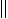PJ
m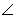QBK = _________
mJNR = 832.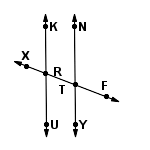KUNY
mXRK = _________
mYTF = 593.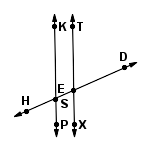KPTX
mPSE = _________
mSET = 1044.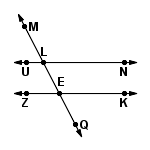UNZK
mNLE = _________
mKEQ = 1175.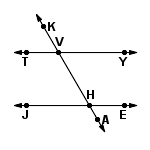TYJE
mTVH = _________
mYVH = 1206.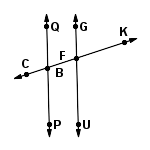QPGU
mCBP = _________
mBFU = 827.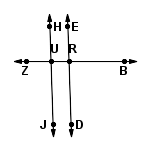HJED
mZUH = _________
mDRB = 808.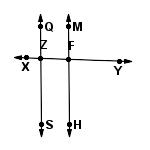QSMH
mZFH = _________
mXZS = 1029 * This is a pre-made sheet.Use the link at the top of the page for a printable page.